2022: SklogWiki celebrates 15 years on-line

# Critical exponents

Critical exponents. Groups of critical exponents form universality classes.

## Reduced distance:$\epsilon$$\epsilon$ is the reduced distance from the critical temperature, i.e.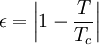$\epsilon = \left| 1 -\frac{T}{T_c}\right|$

Note that this implies a certain symmetry when the critical point is approached from either 'above' or 'below', which is not necessarily the case.

## Heat capacity exponent: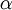$\alpha$

The isochoric heat capacity is given by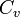$C_v$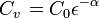$\left. C_v\right.=C_0 \epsilon^{-\alpha}$

Theoretically one has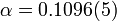$\alpha = 0.1096(5)$ for the three dimensional Ising model, and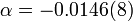$\alpha = -0.0146(8)$ for the three-dimensional XY universality class. Experimentally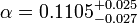$\alpha = 0.1105^{+0.025}_{-0.027}$.

## Magnetic order parameter exponent: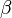$\beta$

The magnetic order parameter,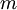$m$ is given by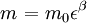$\left. m\right. = m_0 \epsilon^\beta$

Theoretically one has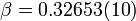$\beta =0.32653(10)$ for the three dimensional Ising model, and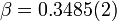$\beta = 0.3485(2)$ for the three-dimensional XY universality class.

## Susceptibility exponent: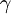$\gamma$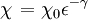$\left. \chi \right. = \chi_0 \epsilon^{-\gamma}$

Theoretically one has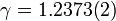$\gamma = 1.2373(2)$ for the three dimensional Ising model, and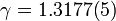$\gamma = 1.3177(5)$ for the three-dimensional XY universality class.

## Correlation length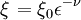$\left. \xi \right.= \xi_0 \epsilon^{-\nu}$

Theoretically one has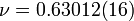$\nu = 0.63012(16)$ for the three dimensional Ising model, and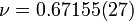$\nu = 0.67155(27)$ for the three-dimensional XY universality class.

## Inequalities

#### Fisher inequality

The Fisher inequality (Eq. 5 )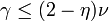$\gamma \le (2-\eta) \nu$

#### Griffiths inequality

The Griffiths inequality (Eq. 3 ):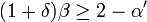$(1+\delta)\beta \ge 2-\alpha'$

#### Josephson inequality

The Josephson inequality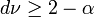$d\nu \ge 2-\alpha$

#### Rushbrooke inequality

The Rushbrooke inequality (Eq. 2 ), based on the work of Essam and Fisher (Eq. 38 ) is given by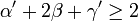$\alpha' + 2\beta + \gamma' \ge 2$.

Using the above-mentioned values one has: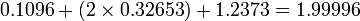$0.1096 + (2\times0.32653) + 1.2373 = 1.99996$

#### Widom inequality

The Widom inequality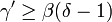$\gamma' \ge \beta(\delta -1)$

## Gamma divergence

When approaching the critical point along the critical isochore (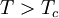$T > T_c$) the divergence is of the form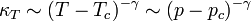$\left. \right. \kappa_T \sim (T-T_c)^{-\gamma} \sim (p-p_c)^{-\gamma}$

where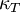$\kappa_T$ is the isothermal compressibility.$\gamma$ is 1.0 for the Van der Waals equation of state, and is usually 1.2 to 1.3.

## Epsilon divergence

When approaching the critical point along the critical isotherm the divergence is of the form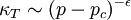$\left. \right. \kappa_T \sim (p-p_c)^{-\epsilon}$

where$\epsilon$ is 2/3 for the Van der Waals equation of state, and is usually 0.75 to 0.8.